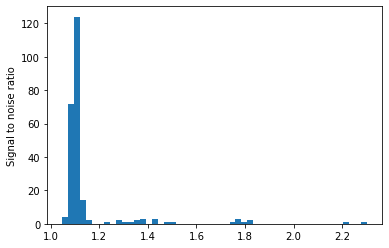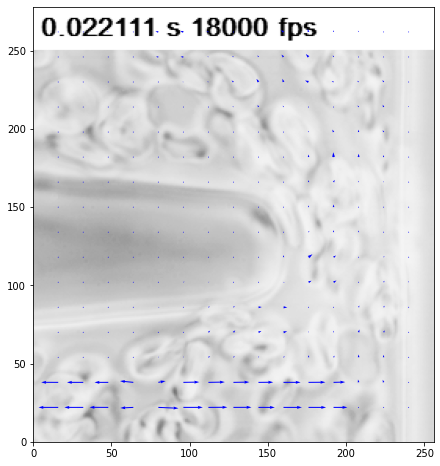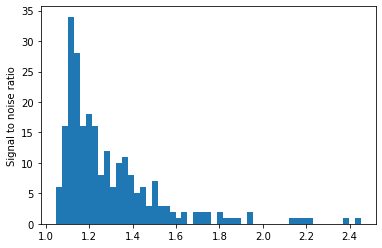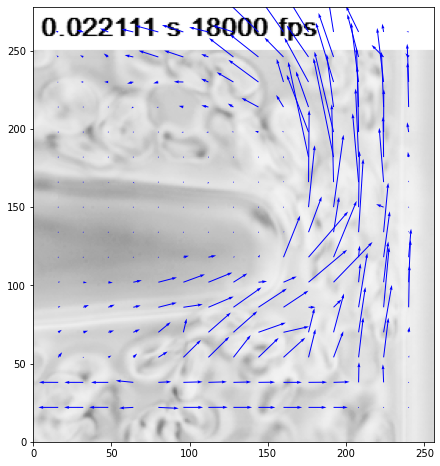# Single pass on complex images, effect of normalized correlation¶

In :
from openpiv import tools, pyprocess, scaling, validation, filters
import numpy as np
import glob
import matplotlib.pyplot as plt
%matplotlib inline

In :
# set of typical parameters
window_size = 32  # pixels 32 x 32 pixels interrogation window, in frame A.
overlap = 16  # overlap is 8 pixels, i.e. 25% of the window
search_size = 32  # pixels 64 x 64 in frame B, avoids some peak locking for
# large displacements

In :
def openpiv_default_run(im1,im2,normalized_correlation=False):
""" default settings for OpenPIV analysis using
extended_search_area_piv algorithm for two images

Inputs:
im1,im2 : str,str = path of two image
"""
frame_a  = tools.imread(im1)
frame_b  = tools.imread(im2)

u, v, sig2noise = pyprocess.extended_search_area_piv(frame_a.astype(np.int32),
frame_b.astype(np.int32),
window_size=window_size,
overlap=overlap,
dt=1,
search_area_size=search_size,
sig2noise_method='peak2peak',
correlation_method='linear',
normalized_correlation=normalized_correlation)
x, y = pyprocess.get_coordinates(frame_a.shape,
search_size,
overlap)
u, v, mask = validation.sig2noise_val(u, v, sig2noise, threshold = 1.0 )
plt.figure()
plt.hist(sig2noise.flatten(),51)
plt.ylabel('Signal to noise ratio')
u, v = filters.replace_outliers( u, v, method='localmean',
max_iter=1, kernel_size=2)
x, y, u, v = scaling.uniform(x, y, u, v, scaling_factor = 1. )
tools.save(x, y, u, v, mask, list_of_images+'.txt' )
fig, ax = plt.subplots(figsize=(8,8))
fig,ax = tools.display_vector_field(list_of_images+'.txt',
on_img=True,image_name=list_of_images,
scaling_factor=1.,
scale=20,
ax=ax)

In :
alist_filter = ['jpg','bmp','png','tif','tiff']

# all test cases in /openpiv/examples/
list_of_files = glob.glob('../test3/*.*')
list_of_files.sort()
list_of_images = [f for f in list_of_files if f[-3:] in alist_filter]
list_of_images.sort()
if len(list_of_images) > 1:
print(list_of_images, list_of_images)
openpiv_default_run(list_of_images,list_of_images,normalized_correlation=False)

../test3/Y4-S3_Camera000398.tif ../test3/Y4-S3_Camera000399.tif

/home/user/miniconda3/envs/openpiv/lib/python3.8/site-packages/numpy/core/fromnumeric.py:3419: RuntimeWarning: Mean of empty slice.
return _methods._mean(a, axis=axis, dtype=dtype,
/home/user/miniconda3/envs/openpiv/lib/python3.8/site-packages/numpy/core/_methods.py:188: RuntimeWarning: invalid value encountered in double_scalars
ret = ret.dtype.type(ret / rcount)In :
if len(list_of_images) > 1:
print(list_of_images, list_of_images)
openpiv_default_run(list_of_images,list_of_images,normalized_correlation=True)

../test3/Y4-S3_Camera000398.tif ../test3/Y4-S3_Camera000399.tifIn [ ]:


In [ ]:


In [ ]: